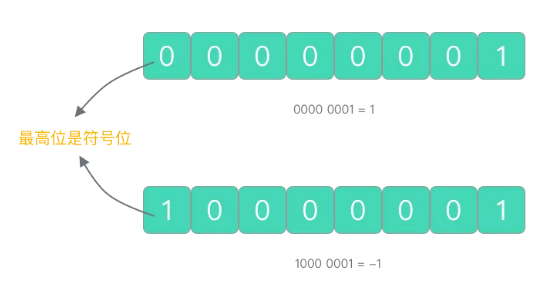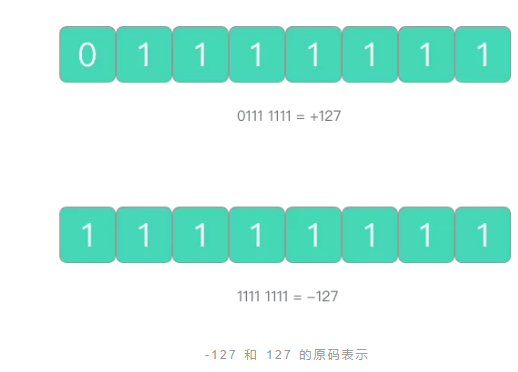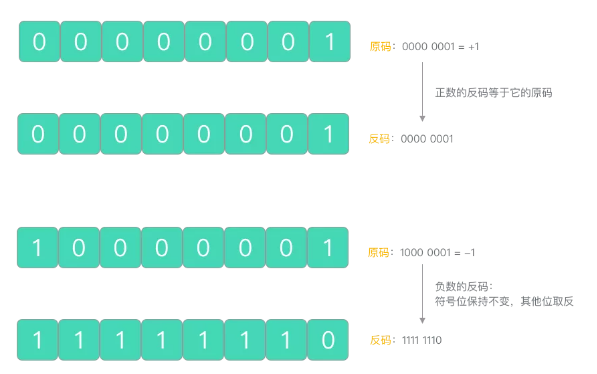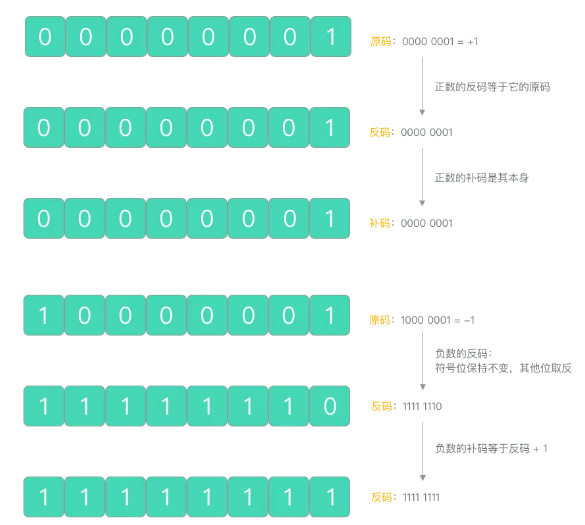2019
12-13

# 深入浅出：举个例子解读原码、反码与补码```package main import "fmt" func main() {
var a int8 = -128         var b = a / -1         fmt.Println(b)
}```

## 有符号整数## 原码、反码和补码

### 原码

• 当二进制数大于 0 时，符号位为 0
• 当二进制数小于 0 时，符号位为 1-127 和 127 的原码表示因此，用这种编码方式表示有符号的 8 位二进制数，它的取值范围是 [1111 1111, 0111 1111]，即 [-127, 127]。如果我们使用原码计算 (+1) + (-1) 会得到什么结果呢？

```(+1) + (-1) =

0000 0001（原码）
+
1000 0001（原码）
=
1000 0010（原码）
=
(-2)```
What? 等于 -2 了？这显然是错误的答案。

### 反码

• 正数的反码等于它的原码
• 负数的反码则保留其原码符号位，然后对其他位进行取反操作```(+1) + (-1) =

0000 0001（反码）
+
1111 1110（反码）
=
1111 1111（反码）
=
(-0)```

### 补码

• 正数的补码是其本身
• 负数的补码是在它的反码基础上加 1```(+1) + (-1) =

0000 0001（补码）
+
1111 1111（补码）
=
0000 0000（补码）
=
(0)```

## -128 的由来

```(-1) + (-127) =

1000 0001（原码）+
1111 1111（原码）
=
1111 1110（反码）+
1000 0000（反码）
=
1111 1111（补码）+
1000 0001（补码）
=
1 1000 0000（补码）
=
1000 0000（丢弃最高位）
=
(-128)```
(-1) + (-127) 的结果正是 -128。但由于 1000 0000  -0 的补码，所以 -128 没有与之对应的原码和反码表示。

## 公布答案

```package main import "fmt" func main() {
var a int8 = -128         var b = a / -1         fmt.Println(b)
}```

## 总结

• int8 表示有符号 8 位整数，它的可表示范围是 [-128, 127]
• 计算机内部使用补码方式表示负数
• 补码解决了 +0  -0 并存的问题，并省下 -0 的表示方法，多表示了一个最低数 -128
• 补码使得所有整数集都能使用同一套加法规则
• 如果发生溢出，多出的高位将被截取